Back to Contents

# Case by Case

In the previous chapter, we used the conditional expression  `if` … `then` … `else`  to define functions whose results depend on their arguments. For some of them we had to nest the conditional expressions one inside another. Programs like this are not terribly easy to read, and expand quickly in size and complexity as the number of cases increases.

OCaml has a nicer way of expressing choices – pattern matching. For example, recall our factorial function: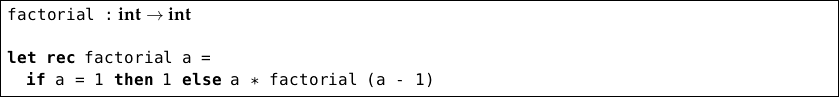We can rewrite this using pattern matching: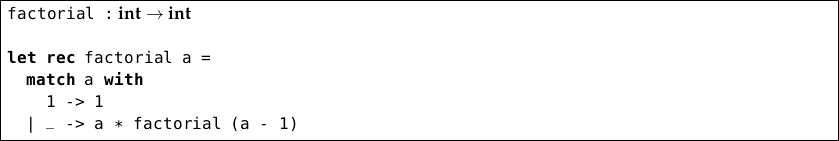We can read this as “See if `a` matches the pattern `1`. If it does, just return `1`. If not, see if it matches the pattern `_`. If it does, the result is `a * factorial (a - 1)`.” The pattern `_` is special – it matches anything. Remember our `isvowel` function from the previous chapter?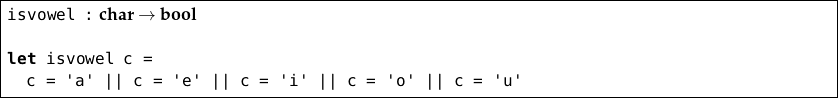Here is how to write it using pattern matching: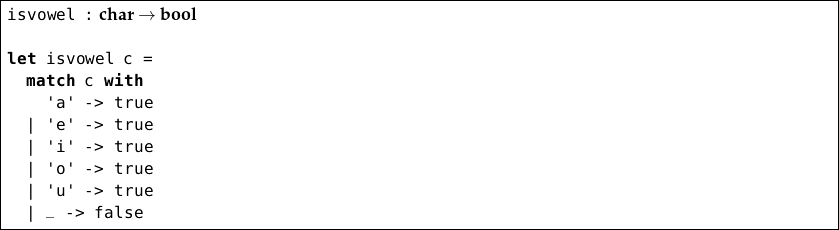If we miss out one or more cases, OCaml will warn us:

` OCaml`

`# let isvowel c =`
`    match c with`
`      ’a’ -> true`
`    | ’e’ -> true`
`    | ’i’ -> true`
`    | ’o’ -> true`
`    | ’u’ -> true;;`
`# Warning 8 [partial-match]: this pattern-matching is not exhaustive.`
`Here is an example of a case that is not matched:`
`'b'`
`val isvowel : char -> bool`

OCaml does not reject the program, because there may be legitimate reasons to miss cases out, but for now we will make sure all our pattern matches are complete. Notice that we had to repeat `true` five times. This would be awkward if the expression to be calculated was more complicated. We can combine patterns like this: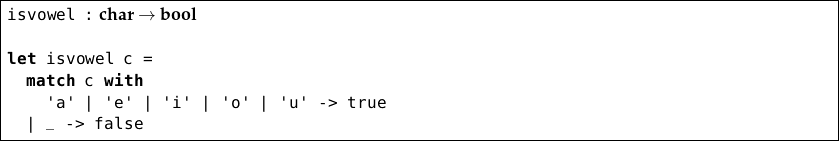Finally, let us rewrite Euclid’s Algorithm from the previous chapter: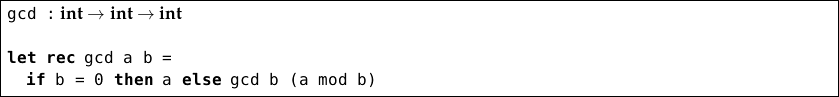Now in pattern matching style: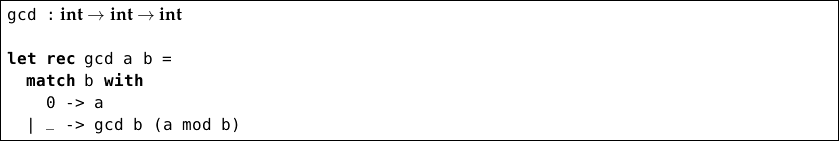The type of a whole  `match`  …  `with` … expression is determined by the types of the expressions on the right hand side of each `->` arrow, all of which must be alike:We use pattern matching whenever it is easier to read and understand than  `if` … `then` … `else`  expressions.

## Questions

1. Rewrite the `not` function from the previous chapter in pattern matching style.

2. Use pattern matching to write a recursive function which, given a positive integer n, returns the sum of all the integers from 1 to n.

3. Use pattern matching to write a function which, given two numbers x and n, computes xn.

4. For each of the previous three questions, comment on whether you think it is easier to read the function with or without pattern matching. How might you expect this to change if the functions were much larger?

5. What does `match`` 1 + 1 ``with`` 2 -> ``match`` 2 + 2 ``with`` 3 -> 4 | 4 -> 5` evaluate to?

6. There is a special pattern `x..y` to denote continuous ranges of characters, for example `’a’..’z’` will match all lowercase letters. Write functions `islower` and `isupper`, each of type char bool, to decide on the case of a given letter.